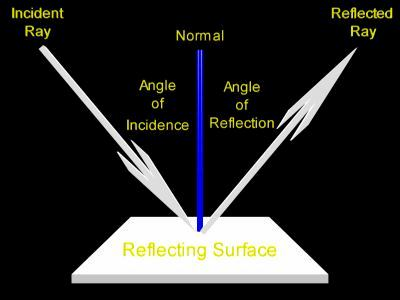# sir please give me all details aboutREFLECTION IN RAY OPTICSSO THAT I CAN SOLVE PROBLEMS OF IT

11 years ago

At first we will look at a simpler reflection, one that happens on a flat surface. Below is a picture of such a situation. Here a single ray of light strikes a surface and is reflected from it.Here are descriptions for the terms in this diagram:

• The ray of light which strikes the surface is called the incident ray.
• The ray of light which leaves the surface is called the reflected ray.
• A line perpendicular to the surface is imagined at the point of reflection. This line is called a normal. In this context the word normal means perpendicular. In the above diagram the normal is colored blue.
• The angle between the incident ray and the normal is called the angle of incidence, or the incident angle.
• The angle between the reflected ray and the normal is called the angle of reflection, or the reflected angle.

Notice that the angle of incidence is equal to the angle of reflection.

The reflection of light is often discussed using phrases such as "a ray of light bounces off of a mirror." This is because when a light ray reflects at the surface of a mirror it follows a path similar in behavior to a pool ball bouncing off of a cushion on a pool table. However unusual it may sound at first, it is not really the best idea to describe the reflection of a light ray using words like bounce. It is better to describe light ray reflection as the turning back of the ray when it encounters the edge of a medium. Light rays, at least at first study, are best not quickly described in terms of particles, say, like pool balls.

Below, in an animated diagram, a light beam reflects at the surface of a flat mirror. This diagram demonstrates the reflective behavior of any single ray of light.

Click on the picture to change the origin of the light ray. Drag slowly.

In the above diagram the reflecting surface is drawn in red, the normal is drawn in blue.